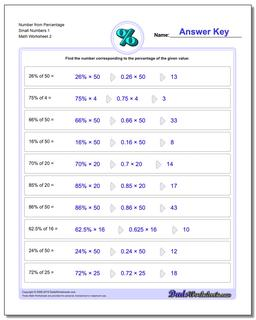# Math Worksheets: Percentages: Percentages: Number from Percentage Small Numbers 1 (Second Worksheet)## Number from Percentage Small Numbers 1 (Second Worksheet)

PropertyValue
DescriptionNumber from Percentage Small Numbers 1: Percent worksheets where students are given a whole number and a percentage, and must calculate the fraction of the whole represented. (Second Worksheet)
Resource TypeWorksheet